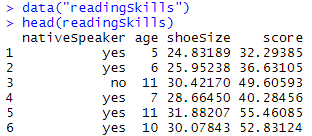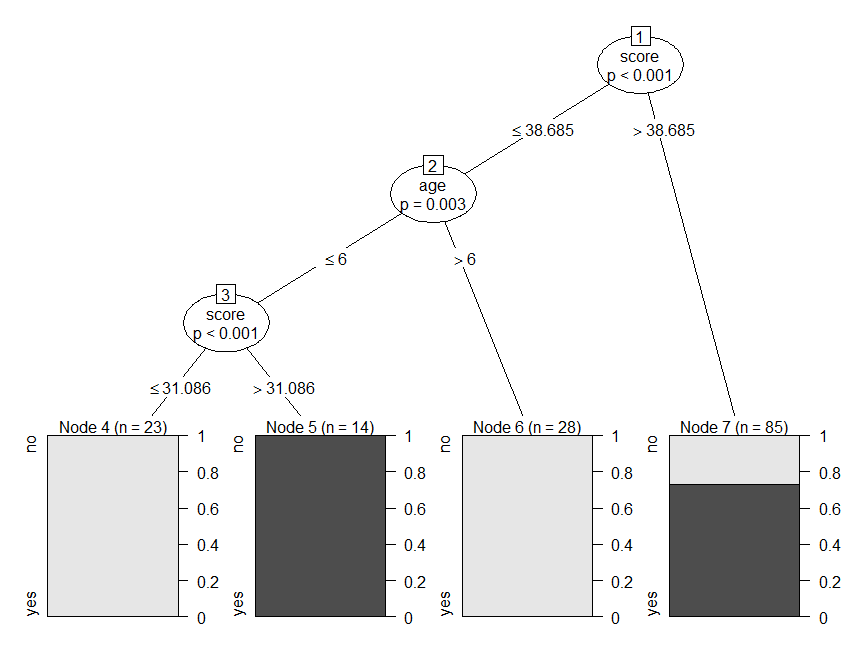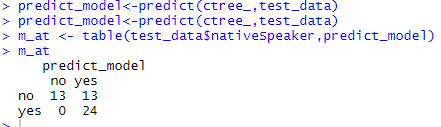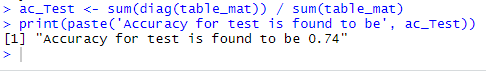GeeksforGeeks App
Open AppBrowser
Continue

# Decision Tree in R Programming

Decision Trees are useful supervised Machine learning algorithms that have the ability to perform both regression and classification tasks. It is characterized by nodes and branches, where the tests on each attribute are represented at the nodes, the outcome of this procedure is represented at the branches and the class labels are represented at the leaf nodes. Hence it uses a tree-like model based on various decisions that are used to compute their probable outcomes. These types of tree-based algorithms are one of the most widely used algorithms due to the fact that these algorithms are easy to interpret and use. Apart from this, the predictive models developed by this algorithm are found to have good stability and a descent accuracy due to which they are very popular

## Types of Decision Trees

• Decision stump: Used for generating a decision tree with just a single split hence also known as a one-level decision tree. It is known for its low predictive performance in most cases due to its simplicity.
• M5: Known for its precise classification accuracy and its ability to work well to a boosted decision tree and small datasets with too much noise.
• ID3(Iterative Dichotomiser 3): One of the core and widely used decision tree algorithms uses a top-down, greedy search approach through the given dataset and selects the best attribute for classifying the given dataset
• C4.5: Also known as the statistical classifier this type of decision tree is derived from its parent ID3. This generates decisions based on a bunch of predictors.
• C5.0: Being the successor of the C4.5 it broadly has two models namely the basic tree and rule-based model, and its nodes can only predict categorical targets.
• CHAID: Expanded as Chi-squared Automatic Interaction Detector, this algorithm basically studies the merging variables to justify the outcome on the dependent variable by structuring a predictive model
• MARS: Expanded as multivariate adaptive regression splines, this algorithm creates a series of piecewise linear models which is used to model irregularities and interactions among variables, they are known for their ability to handle numerical data with greater efficiency.
• Conditional Inference Trees: This is a type of decision tree that uses a conditional inference framework to recursively segregate the response variables, it’s known for its flexibility and strong foundations.
• CART: Expanded as Classification and Regression Trees, the values of the target variables are predicted if they are continuous else the necessary classes are identified if they are categorical.

As it can be seen that there are many types of decision trees but they fall under two main categories based on the kind of target variable, they are:

• Categorical Variable Decision Tree: This refers to the decision trees whose target variables have limited value and belong to a particular group.
• Continuous Variable Decision Tree: This refers to the decision trees whose target variables can take values from a wide range of data types.

## Decision Tree in R Programming Language

Let us consider the scenario where a medical company wants to predict whether a person will die if he is exposed to the Virus. The important factor determining this outcome is the strength of his immune system, but the company doesn’t have this info. Since this is an important variable, a decision tree can be constructed to predict the immune strength based on factors like the sleep cycles, cortisol levels, supplement intaken, nutrients derived from food intake, and so one of the person which is all continuous variables.

### Working of a Decision Tree in R

• Partitioning: It refers to the process of splitting the data set into subsets. The decision of making strategic splits greatly affects the accuracy of the tree. Many algorithms are used by the tree to split a node into sub-nodes which results in an overall increase in the clarity of the node with respect to the target variable. Various Algorithms like the chi-square and Gini index are used for this purpose and the algorithm with the best efficiency is chosen.
• Pruning: This refers to the process wherein the branch nodes are turned into leaf nodes which results in the shortening of the branches of the tree. The essence behind this idea is that overfitting is avoided by simpler trees as most complex classification trees may fit the training data well but do an underwhelming job in classifying new values.
• Selection of the tree: The main goal of this process is to select the smallest tree that fits the data due to the reasons discussed in the pruning section.

### Important factors to consider while selecting the tree in R

• Entropy:
Mainly used to determine the uniformity in the given sample. If the sample is completely uniform then entropy is 0, if it’s uniformly partitioned it is one. The higher the entropy more difficult it becomes to draw conclusions from that information.
• Information Gain:
Statistical property which measures how well training examples are separated based on the target classification. The main idea behind constructing a decision tree is to find an attribute that returns the smallest entropy and the highest information gain. It is basically a measure in the decrease of the total entropy, and it is calculated by computing the total difference between the entropy before split and average entropy after the split of dataset based on the given attribute values.

## R – Decision Tree Example

Let us now examine this concept with the help of an example, which in this case is the most widely used “readingSkills” dataset by visualizing a decision tree for it and examining its accuracy.

## R

 `install.packages``(``'datasets'``)``install.packages``(``'caTools'``)``install.packages``(``'party'``)``install.packages``(``'dplyr'``)``install.packages``(``'magrittr'``)`

## R

 `library``(datasets)``library``(caTools)``library``(party)``library``(dplyr)``library``(magrittr)` `data``(``"readingSkills"``)``head``(readingSkills)`

Output:As you can see clearly there 4 columns nativeSpeaker, age, shoeSize, and score. Thus basically we are going to find out whether a person is a native speaker or not using the other criteria and see the accuracy of the decision tree model developed in doing so.

## R

 `sample_data = ``sample.split``(readingSkills, SplitRatio = 0.8)``train_data <- ``subset``(readingSkills, sample_data == ``TRUE``)``test_data <- ``subset``(readingSkills, sample_data == ``FALSE``)`

Separating data into training and testing sets is an important part of evaluating data mining models. Hence it is separated into training and testing sets. After a model has been processed by using the training set, you test the model by making predictions against the test set. Because the data in the testing set already contains known values for the attribute that you want to predict, it is easy to determine whether the model’s guesses are correct.

## R

 `model<- ``ctree``(nativeSpeaker ~ ., train_data)``plot``(model)`

### The basic syntax for creating a decision tree in R is:

`ctree(formula, data)`

where, formula describes the predictor and response variables and data is the data set used. In this case, nativeSpeaker is the response variable and the other predictor variables are represented by, hence when we plot the model we get the following output.

Output:From the tree, it is clear that those who have a score less than or equal to 31.08 and whose age is less than or equal to 6 are not native speakers and for those whose score is greater than 31.086 under the same criteria, they are found to be native speakers.

## R

 `# testing the people who are native speakers``# and those who are not``predict_model<-``predict``(ctree_, test_data)` `# creates a table to count how many are classified``# as native speakers and how many are not``m_at <- ``table``(test_data\$nativeSpeaker, predict_model)``m_at`

OutputThe model has correctly predicted 13 people to be non-native speakers but classified an additional 13 to be non-native, and the model by analogy has misclassified none of the passengers to be native speakers when actually they are not.

## R

 `ac_Test < - ``sum``(``diag``(table_mat)) / ``sum``(table_mat)``print``(``paste``(``'Accuracy for test is found to be'``, ac_Test))`

Output:Here the accuracy-test from the confusion matrix is calculated and is found to be 0.74. Hence this model is found to predict with an accuracy of 74 %.

## Inference

Thus Decision Trees are very useful algorithms as they are not only used to choose alternatives based on expected values but are also used for the classification of priorities and making predictions. It is up to us to determine the accuracy of using such models in the appropriate applications.

• Easy to understand and interpret.
• Does not require Data normalization
• Doesn’t facilitate the need for scaling of data
• The pre-processing stage requires lesser effort compared to other major algorithms, hence in a way optimizes the given problem